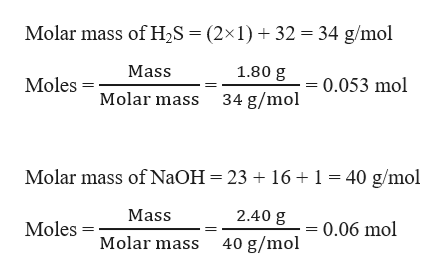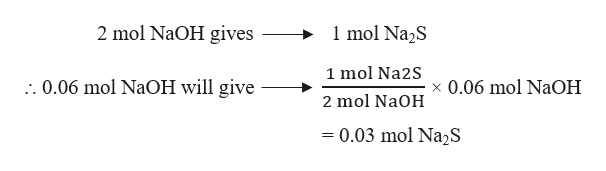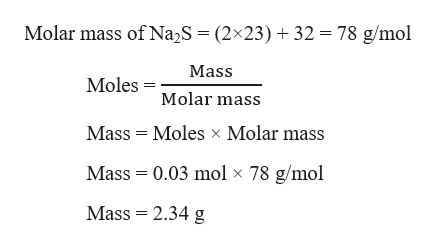# How many grams of sodium sulfide are actually formed if  1.80 g of hydrogen sulfide is bubbled into a solution containing 2.40 g of sodium hydroxide, assuming that the sodium sulfide is made in 93.0 % yield? Hint:  Set up the balanced chemical equation first.  Calculate the theoretical yield, then the actual yield.

Question
31 views

How many grams of sodium sulfide are actually formed if  1.80 g of hydrogen sulfide is bubbled into a solution containing 2.40 g of sodium hydroxide, assuming that the sodium sulfide is made in 93.0 % yield? Hint:  Set up the balanced chemical equation first.  Calculate the theoretical yield, then the actual yield.

check_circle

Step 1

Given,

Mass of hydrogen sulfide, H2S = 1.80 g

Mass of sodium hydroxide, NaOH = 2.40 g

Moles of H2S and NaOH can be calculated as:help_outlineImage TranscriptioncloseMolar mass of H2S = (2x 1)32 34 g/mol 1.80 g Mass 0.053 mol Moles Molar mass 34 g/mol Molar mass of NaOH 23 161 40 g/mol 2.40 g Mass 0.06 mol Moles Molar mass 40 g/mol fullscreen
Step 2

The balanced chemical reaction can be written as:

2 NaOH + H2S → Na2S + 2 H2O

From the reaction, the stoichiometric molar ratio of H2S : NaOH = 1 : 2

And the calculated molar ratio of H2S : NaOH = 1 : 1.13

That means NaOH is the limiting reagent, thus the moles of Na2S will depend on the moles of NaOH. Therefore, moles of Na2S formed can be calculated as:help_outlineImage Transcriptionclose1 mol Na2S 2 mol NaOH gives 1 mol Na2S . 0.06 mol NaOH will give x 0.06 mol NaOH 2 mol NaOH =0.03 mol N22S fullscreen
Step 3

Grams of Na2S can be calc...help_outlineImage Transcriptionclose78 g/mol Molar mass of Na2S = (2x23) 32 Mass MolesMolar mass Mass Moles x Molar mass Mass 0.03 mol x 78 g/mol Mass 2.34 g fullscreen

### Want to see the full answer?

See Solution

#### Want to see this answer and more?

Solutions are written by subject experts who are available 24/7. Questions are typically answered within 1 hour.*

See Solution
*Response times may vary by subject and question.
Tagged in

### Chemistry